Quadrilaterals topic is one of the most crucial areas for the Quantitative Section of the CAT. Several questions are asked from the related concepts and so, the candidates are suggested to get well acquainted with the topic. Being able to solve the quadrilateral questions can ensure decent score in the exam.

Introduction:

Quadrilaterals are two-dimensional four-sided polygons on a plane. They have four internal angles and the sum of measures of all angles in a quadrilateral is 360°.

Area of a quadrilateral = ½ × one of the diagonals×sum of the perpendicular drawn to that diagonal from the opposite vertices.

Proof:

A quadrilateral can be assumed to be of 2 triangles,  ADC and ABC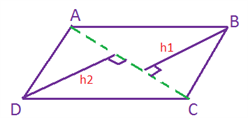Area of △ABC= ½ h1 x AC

Area of △ADC= ½ h2 x AC

Thus, area of the quadrilateral = 1/2 (h1+h2) x AC.

1) Parallelogram

In a parallelogram, the opposite sides are equal and the opposite angles are equal. Opposite sides are parallel.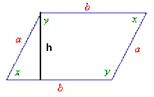• Properties Of Parallelogram:
*Diagonals bisect each other.
*Straight lines joining the midpoints of the adjacent sides of any quadrilateral form a Parallelogram.

*Opposite angles are equal.

*Sum of any two adjacent angles= 1800*Area= Basexheight(h).

*Area of a triangle having the same base and the same height = ½ (Area of the parallelogram).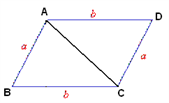Area of △ABC= ½ Area of ABCD

ILLUSTRATION:

Question: One angle of a parallelogram measures (2a+b)o. another angle of this parallelogram ( not the opposite angle) measures (a+2b)o. find a+b.

Solution:

Adjacent angles in a parallelogram are supplementary. Hence 2a+b+a+2b=180o Hence 3(a+b)=180o =>a+b=60o

2) Rectangles

A rectangle is a special case of a parallelogram. The opposite sides are equal and all angles are right angles (i.e. 900).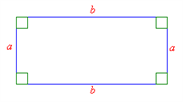• Properties of Rectangles:

*Opposite sides are parallel and congruent

*Each angle= 900

*Diagonals are congruent and bisect each other (not at right angles)

* Perimeter= 2(l+b) (l= length and b= breadth)

* Area= l*b

*Diagonal= v((l2+b2 ) )

*A quadrilateral formed by the points of intersection of the angle bisectors of a parallelogram is a rectangle

If P is any point inside a rectangle, then PA2+PC2=PB2+PD2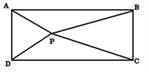3) Rhombus

A rhombus is a special case of a parallelogram with four equal sides.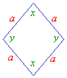PROPERTIES OF A RHOMBUS

* Opposite sides are parallel

*All sides are congruent(equal)

* Opposite angles are congruent

* Diagonals bisect each other at right angles

*Area= ½ *product of diagonals.

*Side2=(1/2Diagonal)2 + (1/2 other Diagonal)2

Squares

A square is a special case of a rectangle in which the lengths of all sides are equal.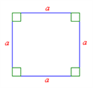PROPERTIES OF A SQUARE

*All sides are congruent

* Diagonals are congruent

* Diagonals bisect at right angles

* All angles are 900

* Perimeter=4*side *Area =side2=1/2(diagonal)2

Trapezium/Trapezoid

A trapezium is a four-sided polygon that has exactly two sides parallel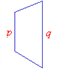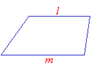For example, in these two trapeziums, l is parallel to m and p is parallel to q.

Properties of a trapezium.

Exactly two sides are parallel

Median= ½ *(sum of parallel sides)

Area=1/2*(sum of parallel sides)*(height) It is an isosceles trapezium if the non-parallel sides are congruent (AB=CD)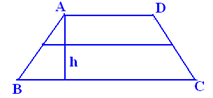ILLUSTRATIONS

Question: PQRS is an isosceles trapezium with PQ=10 and RS=6. The length of TU, the altitude=8. Find the perimeter of PQRS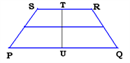Solution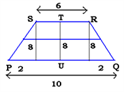PS= v(22+82 )= v68= 2v17

Hence RQ= 2v17 Perimeter = 10+6+4v17= 16+4v17

Kite

Properties of a kite.

*It has two pairs of equal and adjacent sides

*Diagonals intersect at right angles

*Longer diagonal bisects the shorter diagonal

*Area=1/2 * (product of diagonals)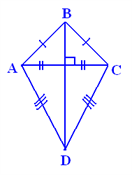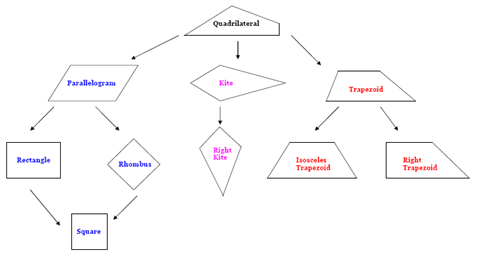1. If you move down the chart then use Sometimes.

2. If you move up the chart then use Always.

3. If you move across the chart then use Never.

i.e. Every square is a Rectangle because you are moving up the chart, but a rectangle may sometimes be a square because you are moving down the chart Is a quadrilateral a kite?The answer is Sometimes because you moved down the chart

Let us now look at a quick summary of the properties of different quadrilaterals in a similar chart.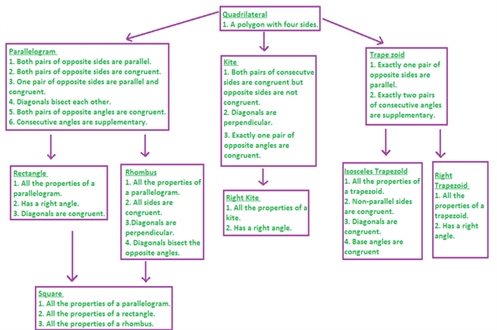PENTAGONS

A polygon of 5 sides is called a pentagon. In a regular pentagon, each interior angle= (2n-4)× 90/n= (2×5-4)(90 )/5= 108o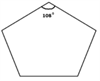HEXAGONS

A convex polygon of six sides is called a hexagon. In a regular hexagon, each interior angle= 1200

Area of a regular hexagon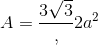Six equilateral triangles make a hexagon (learn how to solve area based questions very fast based on this logic in Graphical Division, explained later in this chapter)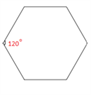Get acquainted with more such important topics along with various engaging video lessons at Byju’s. ALso, know the latest CAT 2017 Notification and CAT syllabus to prepare a proper strategy for the exam